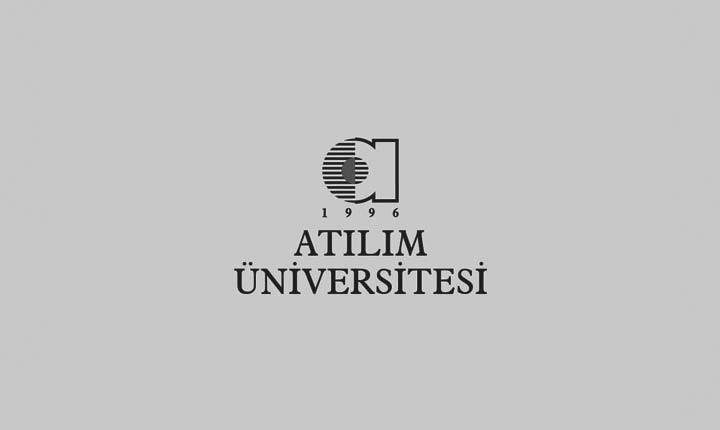# Fen - EdebiyatOrdinary Differential Equations : Problem Book With Solutions ; Rajeh Eid.

Ankara: 2005.

128 p. ; 30 cm.

(Atılım University Publications; 16. Faculty of Arts & Science; 3)

QA 372 EID 2005

ISBN: 9756707143

FİYAT: 15 TL

İÇİNDEKİLER

1. First order ordinary differential equations

2.  Higher  order linear ordinary differential equations

3. Series solutions of second order linear ordinary differential equations including legendre and bessel equations

4. The leplace transform

5. Systems of linear ordinary differential equations.